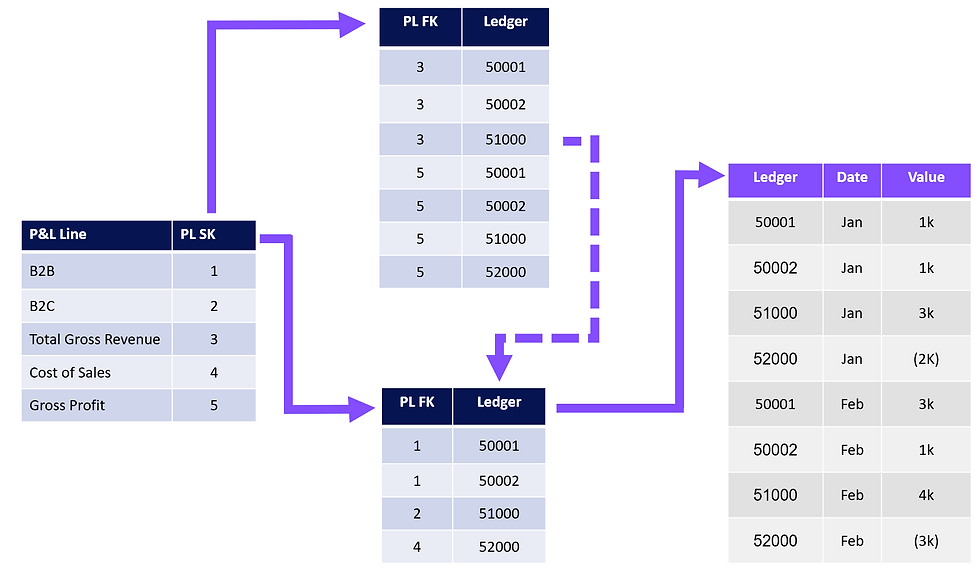top of page

# Deviating from Star Schema Principles

Updated: Feb 13

#### A finance user caseThe Star Schema methodology is the application of dimensional modelling techniques to a relational database. This includes not only simple star shaped models, but a wide range of techniques such as snowflaking or factless fact tables. These principles should (almost always) be adopted in Power BI (a relational-like database). This article discusses one of the exceptions; dealing with subtotals.

#### The Problem: Why is a Star Schema not able to produce Financial Statements

Suppose we wish to produce the following:A typical Star Schema (with snowflaking) for this data would look like the below:The sum of "Value" grouped by "P&L line" results in the below table:This doesn't provide an adequate solution as the required subtotals "Total Gross Revenue" and "Gross Profit" are not present.

#### Solution

The advised approach is to create 2 calculations:

• The first calculation sums the Ledger values following the path (1) & (4) as per a usual star schema

• The second calculation sums the Ledger values following the path (2), (3) & (4)This second calculation deviates from Star Schema methodology; relationship (3) is a many-to-many virtual relationship managed through the use of TREATAS. The below table shows the result of both calculations:The 2 calculations are then added together to achieve the desired result:#### Key Benefits of this Approach

1. Native integration with Excel where Pivot tables and formulas can point at the Power BI API resulting in a single-source of the truth across various excel files and Power BI reports

2. Other custom totals, like call out numbers at the bottom of financial statements, can be created - i.e., if below "Earnings Per Share" (EPS) the desire is to show "Full Time Equivalent" (FTE) staff costs this can be achieved

3. Percentages can be created - i.e., Gross Profit percentage - from any combination of sum or subtotal and we can use calculation group formatting strings to format these appropriately in Power BI and any Excel pivot tables or formulas

4. Multiple layouts can be handled - i.e., a senior (more consolidated) view of financial results

5. Performant over large volumes of data - see 1 billion row example

6. Reports can be amended by changing the inputs - no need to re-write DAX

7. Can scale to work with 3-way Financial Reporting

#### Alternative Approaches

There are, of course, alternatives to this approach. The 4 main alternatives are:

1. Duplicating records in the fact

2. DAX for each subtotal

3. Custom Visuals

Options 1 & 2 are included for completeness and are not advised; these violate redundancy principles and become cumbersome to manage, respectively.

Custom visuals are a valid solution and are vital in cases where the formatting options in native Power BI visuals are insufficient. However, a model is still required. Therefore, I would recommend the modelling approach outlined alongside a custom visual. This is because if you allow the custom visual to contain your logic for subtotals you surrender control to the visual (and whomever developed it) for your business logic. Generally, it's better to maintain your logic upstream in your database; this is in line with Roche's maxim of data transformation.

The many-to-many approach is also a valid solution. This also breaks Star Schema principles by creating a many-to-many bridge table. The main advantage of this is the DAX is very simple; a sum will work for subtotals and the sum of ledgers. If you don't have to worry about percentages (i.e., Gross Profit percentage), call out numbers (i.e., FTE) or custom calculations (i.e., EPS) this is a valid solution.

#### Conclusion

Despite breaking Star Schema principles, the model outlined is a valid and performant method for developing financial statements. To learn more and build out your financial solution, join over 1,000 people who have taken the free course on Power BI P&L fundamentals